TWiki >Rise Web>ClimbingRobot > ForceSensingAnkle>SecondPrototype (30 Aug 2005, YongLaePark)

## FSA Prototype 2 Fabrication and Test

Physical dimensions of the second prototype is the same as those of the first prototype. The main differences are:

• Semiconductor strain gages are embedded to the load cell instead of foil gages.
• Three axial forces can be measured at the same time.

• Figure 1: Prototyp 2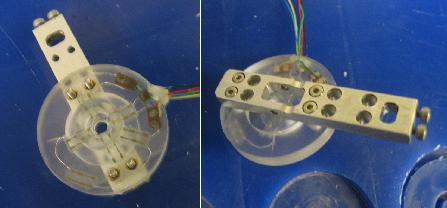### Fabrication

The second prototype was fabricated using SDM process. Figure 2 and 3 show the fabricaing process.

• Figure 2: Strain Gage Placement and Wiring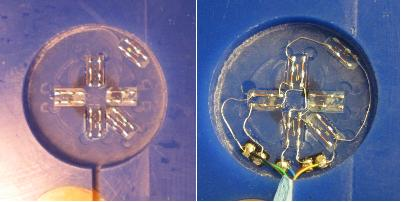• Figure 3: Polyurethane Pouring and Curing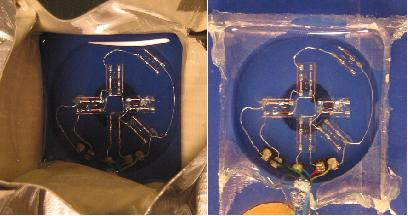### Calibration Test

Force calibration tests were conducted in all three axial forces using a scale and a height gage. Four same tests were conducted for each aixal force test, and all are force loading-unloading tests to check the hysterisis too.

#### Normal Force Test (z-axis)

• Figure 4: Test Setup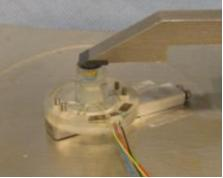• Figure 5: Test Output Plots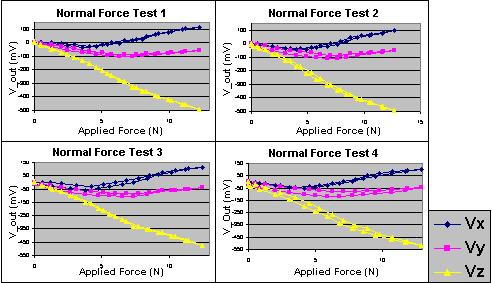• Figure 6: Combined Output Plot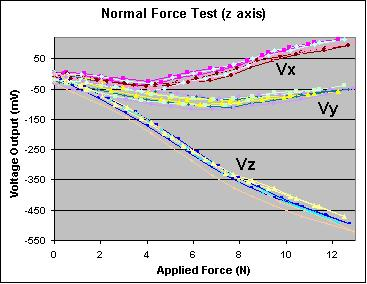#### Side Force Test (x-axis)

• Figure 7: Test Setup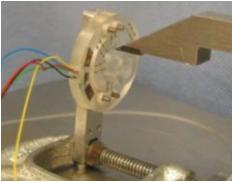• Figure 8: Test Output Plots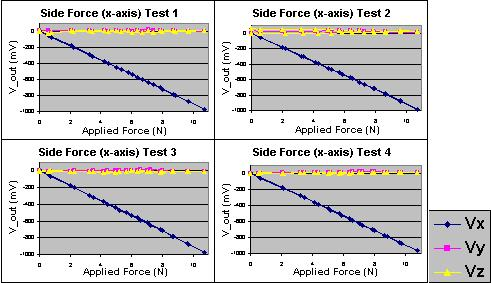• Figure 9: Combined Output Plot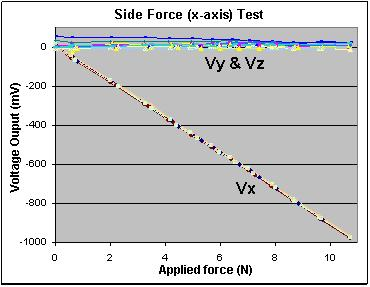#### Up-down Force Test (y-axis)

• Figure 10: Test Setup• Figure 11: Test Output Plots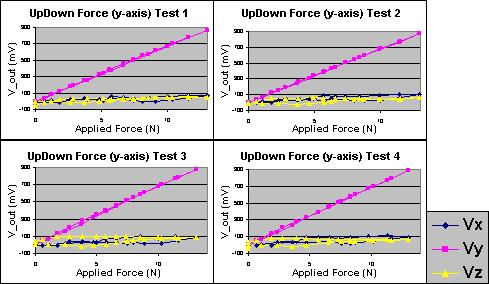• Figure 12: Combined Output Plot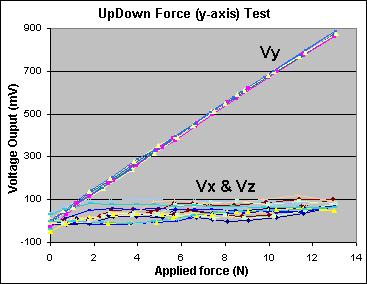For detailed test data and plots, original excel files are attached.

-- YongLaePark - 30 Aug 2005

Copyright &© by the contributing authors. All material on this collaboration platform is the property of the contributing authors.
Ideas, requests, problems regarding TWiki? Send feedback